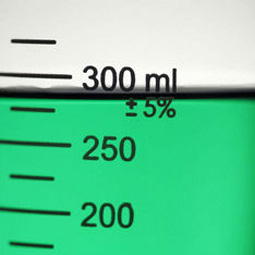# Measured parametersUroflowgram is estimated on the following factors:

• voided volume;
• average volume flow rate;
• maximal volume flow rate;
• uroflow index;
• flow acceleration;
• flow time;
• voiding time;
• time of maximum flow;
• waiting time;
• character of the uroflow graph.

Voided volume (V) — a volume of the urine discharged through an external urethral opening during study. If the urine volumes are from 150 to 500 ml the study results will be more authentic. Dimension — ml.

Flow time (T) — time interval when voiding occurs, without intervals between the separate micturitions. Dimension — s.

Voiding time — time interval from the beginning to the end of voiding, including intervals between separate micturitions. Dimension — s.

Average flow rate (Qavg) — ratio of the discharged voiding volume in milliliters to the flow time in seconds. The most often it is used as the whole uroflow graph index. Dimension — ml/s.

Maximal flow rate (Qmax) — the maximum voiding volume discharged through the external urethral opening per time unit. The given index is defined on uroflow graph as its maximum value. Dimension — ml/s.

Uroflow index (UI) — the value calculated from Siroky nomogram mathematical approximation. Uroflow index allows estimating the urodynamic without dependence on micturition’s volume.
The values UI>1.1 should be considered as normal.
At UI=0.8-1.1 it is necessary to carry out further patient’s examination.
Values UI<0,8 shows an undoubted pathology.
Dimension — 1/s.

Time to maximum flow rate (TQmax) — time interval from the voiding beginning to the maximum volume rate. The given index depends on maximum flow rate and discharged urea volume. Dimension — s.

Waiting time (Tw) — time interval from study beginning to the actual voiding one. Dimension — s.

Character of the uroflow graph (uroflowgram) — there can be observed different types of uroflowgram at various voiding dysfunctions. In typical cases the voiding graph can indicate clear abnormality but it never can be diagnostically specific. Irregular graphs can be caused by an insufficient activity of detrusor, abdominal wall tension, bladderptosia bending of urethra, sphincter dyssynergia or psychological inhibition.
More information about the different types of uroflow graphs can be obtained in the Uroflow study repository section.

Flow-K is equipped with nomograms constructing ability.
Uroflow nomograms represent themselves as the generalization of the experimental data, received at uroflow study of considerable number of patients. Nomograms allow visual estimating of patient’s voiding quality without dependence from voided volume and the patient’s age. Nomogram itself represents as the graphs of 2 variables relation: maximum volume flow rate and voiding volume, or average volume flow rate and voiding volume. Some similar graphs are built. Each graph corresponds to a certain percentile – percent number. For example, if the graph is marked as 5 %, it means, that the flow rate and the points corresponding to these rates, are placed under the given graph, are observed at 5% of patients.
When the point, corresponding to the uroflow parameters, is below the 50% graph, the further examination is of a great importance. If the point is below the 5% graph, it indicates on an unconditional pathology.